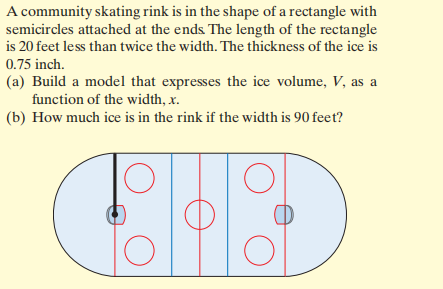### ¿Todavía tienes preguntas de matemáticas?

Pregunte a nuestros tutores expertos
Algebra
PreguntaA community skating rink is in the shape of a rectangle with semicircles attached at the ends The length of the rectangle is $$20$$ feet less than twice the width. The thickness of the ice is $$0.75$$ inch.

(a) Build a model that expresses the ice volume, $$V$$ , as a function of the width, $$x$$ .

(b) How much ice is in the rink if the width is $$90$$ feet?

$$\left. \begin{array} { l l } { \text { (a) } V ( x ) = \frac { x ^ { 2 } } { 8 } - \frac { 5 x } { 4 } + \frac { \pi x ^ { 2 } } { 64 } } & { \text { (b) } 1297.61 ft ^ { 3 } } \end{array} \right.$$